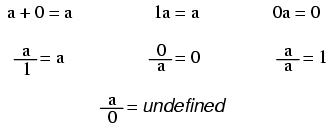# Learning Electronics

Learn to build electronic circuits

# Basic identitiesNote: while division by zero is popularly thought to be equal to infinity, this is not technically true. In some practical applications it may be helpful to think the result of such a fraction approaching positive infinity as a positive denominator approaches zero (imagine calculating current I=E/R in a circuit with resistance approaching zero -- current would approach infinity), but the actual fraction of anything divided by zero is undefined in the scope of either real or complex numbers.